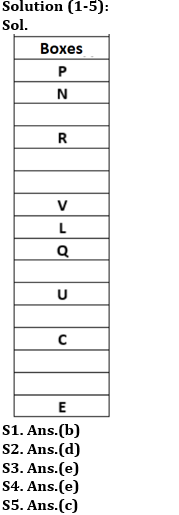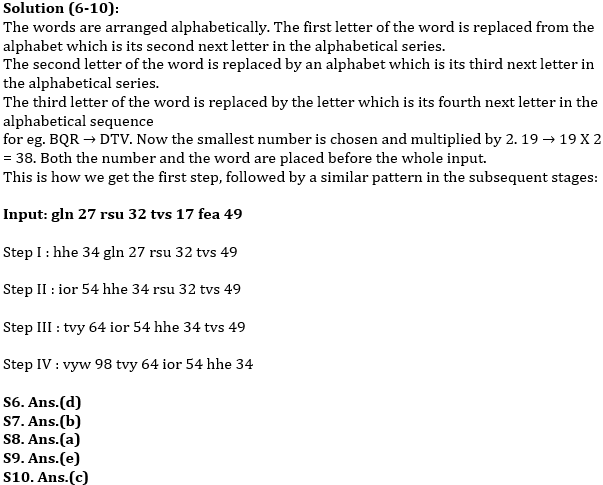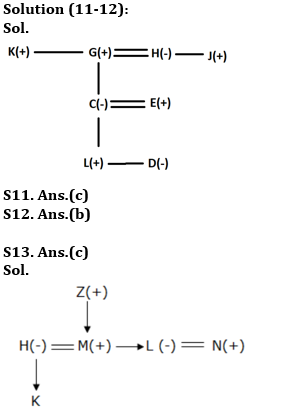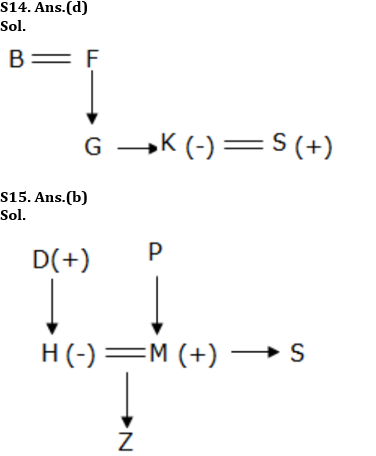Latest Banking jobs   »

# Reasoning Ability Quiz For RBI Assistant/ ESIC UDC Mains 2022- 4th April

Directions (1-5): Study the following information carefully and answer the given questions.
Uncertain numbers of boxes are arranged in a stack from top to bottom but not necessarily in the same order. Box R is not kept adjacent to Box V. Box Q is kept immediately below Box L. Only one box is kept between Box C and Box U. Box R is kept three places below Box P. Box N is kept immediately below Box P. Box L is kept between Box V and Box E. Box C is kept three places above Box E. As many boxes kept between Box C and Box Q as between Box L and Box R. More than two boxes are kept between Box V and Box N. Three boxes kept between Box U and Box V where Box V is kept above Box U. Box L is placed above box U.

Q1. How many minimum number of boxes are kept in the stack?
(a) 12
(b) 16
(c) 13
(d) 14
(e) 15

Q2. Which of the following box are kept immediate above Box L?
(a) N
(b) C
(c) E
(d) V
(e) R

Q3. How many boxes are kept between Box Q and Box R?
(a) One
(b) Three
(c) Two
(d) None
(e) More than three

Q4. Which of the following is/are true with respect to the Box N?
1. Four boxes are kept between Box N and Box V.
2. As many boxes above Box N as below the box which is kept immediately above E
3. Box U is kept above Box Q.
(a) Only (1)
(b) Only (1) and (3)
(c) All (1), (2) and (3)
(d) Only (2) and (3)
(e) Only (1) and (2)

Q5. Which of the following is false with respect to the given arrangement?
(a) Box which is kept two places below Box P is kept third from the top of the stack.
(b) Box R is kept above Box Q.
(c) Two boxes are kept between the box which is kept immediately below Box L and Box C
(d) Box V is kept adjacent to Box L.
(e) None of these

Directions (6-10): Study the following information carefully and answer the given questions. When a word and number arrangement machine is given an input line of words and numbers, it arranges them following a rule. The following is an illustration of Input and rearrangement. (All numbers are of two digits).

Input: fdk 19 bqr 43 teh 21 mop 42

Step I: dtv 38 fdk 43 teh 21 mop 42

Step II: hgo 42 dtv 38 43 teh mop 42

Step III: ort 84 hgo 42 dtv 38 43 teh

Step IV: vhl 86 ort 84 hgo 42 dtv 38

Step IV is the last step for above input.

Find out appropriate steps for the input

Input: Input: gln 27 rsu 32 tvs 17 fea 49

Answer the following questions based on the above information.

Q6. Which is the smallest number in step-IV?
(a) 64
(b) 17
(c) 27
(d) 34
(e) None of these

Q7. Which element is not the part of arrangement?
(a) ior
(b) hee
(c) vyw
(d) hhe
(e) None of these

Q8. “64 ior 54” first appears in which step?
(a) Step III
(b) Step II
(c) Step IV
(d) Step V
(e) None of these

Q9. How many elements are there between ‘98’ and ‘34’ in step IV?
(a) 4
(b) 2
(c) 1
(d) 0
(e) None of these

Q10. Which of the following step is Step IV?
(a) tvs 64 ior 54 hhe 34 vyw 49
(b) hhe 34 gln 27 ior 32 vyw 49
(c) vyw 98 tvy 64 ior 54 hhe 34
(d) vyw 98 ior 64 tvs 34 hhe 54
(e) None of these

Directions (11-12): Study the following information carefully and answer the given questions.
In a family, there are eight members – C, D, E, G, H, J, L and K. C is the wife of E and mother of L. D is the sister of L. K is the uncle of C and brother in law of H. J is the brother of H. L is the grandson of H. H is not a male member. E has one daughter. H is married and she has no sister. K is unmarried.

Q11. Who among the following is father of C?
(a) K
(b) J
(c) G
(d) D
(e) None of these

Q12. What is the relation of K with respect to G?
(a) Son
(b) Brother
(c) Sister
(d) Either (c) or (b)
(e) Father in law

Directions (13-15): These questions are based on the following information.
If R£B means R is parent of B
R&B means R is child of B
R%B means R is siblings of B
R*B means R is married to B
R/B means R is father in law of B
R+B means R is daughter in law of B
R-B means R is brother in law of B

Q13. If K&H+Z/N-M*H, then how L is related to K, if L is the only daughter of Z?
(a) Maternal Uncle
(b) Maternal Aunt
(c) Paternal Aunt
(d) Paternal Uncle
(e) None of these

Q14. If B*F£G%K*S-G, then how S is related to B?
(a) Daughter in law
(b) Son
(c) Daughter
(d) Son in law
(e) None of these

Q15. If D/M£Z&H+P£S, then how H is related to S?
(a) Brother
(b) Sister in law
(c) Brother in law
(d) Cannot be determined
(e) Sister

Solutions